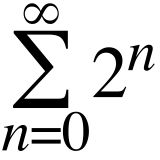# 0.999… equals one.

But you knew that, right? Check out the Wikipedia article about different proofs that 0.999… equals 1. It’s really quite interesting to see the wildly different methods that can be used to arrive at the same conclusion. While I’m partial to the Cauchy sequence version, the first of the proofs is my favorite, beautiful in its simplicity:

0.333…. = 1/3

3* 0.333… = 3 * (1/3)

0.999… = (3*1)/3 = 3/3 = 1

Q.E.D. =)

# Cool Math TrickHere is a cool math trick that shows that the sum of an infinite number of positive integers is equal to negative one.